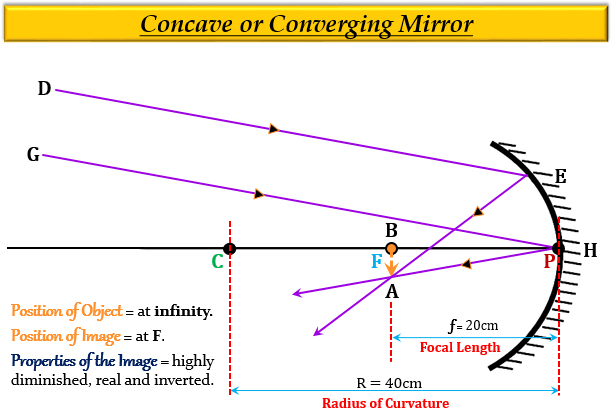# An object is placed at a large distance in front of a concave mirror of radius of curvature 40 cm. The image will be formed in front of the mirror at a distance:(a) 20 cm (b) 30 cm (c) 40 cm (d) 50 cm

(c) 20 cm

Explanation

When an object is placed at a large distance in front of a concave mirror of a radius of curvature $(R)$ 40 cm,  it means the object is at infinity.

And, we know that when the object is at infinity then the image is formed at the focus.

Here, the image will be formed in front of the mirror at a distance of 20 cm, which means that the focal length (distance of focus from the pole) is 20 cm.

This is because the radius of curvature is equal to twice the focal length $(R=2f)$, or the focal length is half of the radius of curvature $(f=\frac {R}{2})$.

Hence, here the focal length $(f)$ is given as- $\frac {40}{2}$ = $20cm$Updated on: 10-Oct-2022

1K+ Views